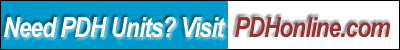Machine Design Excel Calculations

John Andrew, P.E.

Course Outline

This 8 hour online course defines the design equations of several basic machine components resulting in Excel print-outs of design parameters and calculated results. Topics include: Power Transmission Shafting, Straight and Curved Beams, Couplings, Power Screw Actuators, Hydraulic Actuators-Pumps-Motors, Disc and Shoe Brakes, Spur and Worm Gears.

The course includes a multiple-choice quiz at the end, which is designed to enhance the understanding of the course materials.

Learning Objective

At the conclusion of this course the student will:

• Have a spread sheet useful for machine design calculations;
• Adjust machine dimensions to meet design specifications;
• Document design calculations in a spread sheet format;
• Calculate stress and deflections of machine elements;
• Analyze power transmission shafting;
• Design rigid couplings;
• Determine hub and shaft key dimensions;
• Calculate power screw actuator lifting capacity;
• Find brake torque capacity;
• Verify V-belt power transmitting values;
• Optimize spur gear dimensions;
• Calculate the strength and limits of worm gears;
• Determine machine vibration frequencies;
• Analyze forced and damped vibration amplitudes;
• Calculate the critical speed of a shaft with an overhanging load;
• Evaluate cantilever and beam deflections and natural frequencies;
• Determine rectangular and circular plate deflections and natural frequencies;
• Apply static and dynamic balance masses to rotating shafts;
• Calculate shock impulse accelerations and deflections;
• Document engineering calculations in a spread sheet format;
• Create spread sheets for more engineering calculations;
• Be able to employ Excel's Goal Seek tool to optimize designs;
• Use Excel's "Solver" to determine system minimum frequency; and
• Use Excel's "Solver" to solve simultaneous equations.

Intended Audience

The information will be especially useful to students, engineers, and innovators who need tools for creative machine design.

Benefit to Attendees

Attendee of this course will be able to calculate and optimize variables in a range of machine design projects.

Course Introduction

Excel spread sheets are used to calculate and optimize machine design parameters. Machine design calculations have been done with a calculator and written by hand with pen or pencil. Optimizing design values with a calculator is difficult and time consuming. Quite often it is impossible to read and check these documents. As time passes it becomes more difficult to use these documents to make improvements to existing designs.

Course Content

You are required to study the Excel Spreadsheet Machine Design Excel Calculations. This is the only srudy materials for this course.

DISCLAMER: "This spreadsheet is provided for illustrative teaching purpose only, and is not intended for use in any specific project. Anyone making use of theinformation contained in this spreadsheet does so at his/her own risk and assumes any and all resulting liability arising therefrom."

Course Summary

This course provides Excel spread sheets to calculate a range of basic machine design parameters. All variables can be adjusted to optimize design goals. Excel print-outs of design parameters and calculated results provide easy to read documentation for checking and future improvements. Topics include: Power Transmission Shafting, Straight and Curved Beams, Couplings, Power Screw Actuators, Brakes, Spur and Worm Gears.

For additional technical information related to this subject, please visit the following websites or web pages:

430,000 machine items are described and priced at: http://www.mcmaster.com